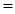Chapter 5, Problem 107SCQ

Chapter
Section
Textbook Problem

You want to heat the air in your house with natural gas (CH4). Assume your house has 275 m2 (about 2800 ft2) of floor area and that the ceilings are 2.50 m from the floors. The air in the house has a molar heat capacity of 29.1 J/mol · K. (The number of moles of air in the house can be found by assuming that the average molar mass of air is 28.9 g/mol and that the density of air at these temperatures is 1.22 g/L.) What mass of methane do you have to burn to heat the air from 15.0 °C to 22.0 °C?

Interpretation Introduction

Interpretation:

The mass of methane to burn to heat the air has to be calculated.

Concept Introduction:

Heat energy required to raise the temperature of 1g of substance by 1K.Energy gained or lost can be calculated using the below equation.

q=C×m×ΔT

Where, q= energy gained or lost for a given mass of substance (m), C =specific heat capacity,ΔT= change in temperature.

The standard molar enthalpy of formation is the enthalpy change ΔfH0 is the enthalpy change for the formation of 1mol a compound directly from its component elements in their standard states. And is given by

ΔrH0ΣnΔfH0(products)-ΣnΔfH0(reactants)

Explanation

To calculate the volume of air

Given,

Density of air=1.22g/L

Volume=275m2×2.50m×1000L1m3×1.22gair1Lair×1mol28.9gair

Volume=2.90×104mol

Substitute the values in,q=C×m×ΔT, as

Specific heat capacity is 29.1J/mol.K

Change in temperature is 7K

q=2.90×104×29.1J/molK×7K= 5.9×106J

The reaction is CH4+2O22H2O+CO2

Substitute the values in ΔrH0

Still sussing out bartleby?

Check out a sample textbook solution.

See a sample solution

The Solution to Your Study Problems

Bartleby provides explanations to thousands of textbook problems written by our experts, many with advanced degrees!

Get Started

recognize common strong acids and bases.

Chemistry for Engineering Students

What is a fatty acid micelle?

Organic And Biological Chemistry

True or false? Ribosomes are only found in eukaryotes.

Biology: The Unity and Diversity of Life (MindTap Course List)